# 比例中项

……与射影定理

## 比例中项

 a = x x b

"a 比 x 等于 x 比 b"

 a = x x bab = x xab  =  x2

x  =  √(ab)

### 例子：2 和 18 的比例中项是多少？

 2 = x x 18

"2 比 x 等于 x 比 18"

x = √(2×18) = √(36) = 6

 2 = 6 6 18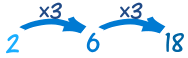（它也是这两个数的几何平均数。）

### 例子：5 和 500 的比例中项是多少？

x = √(5×500)

x = √(2500) = 50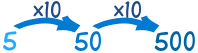## 直角三角形

• 把直角三角形横放，斜边向下
• 在斜边与对角画高线
• 高线把三角形分成两个三角形，对不对？

## 射影定理――第一部分（也称弦高定理）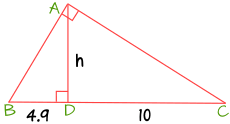左 = 高 高 右

 4.9 = h h 10

h2 = 4.9 × 10 = 49
h = √49 = 7

## 射影定理――第二部分和### 例子：求 x（直角边 AB 的长度）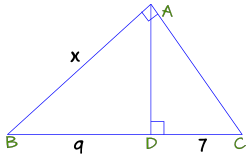斜边 = 直角边 直角边 射影

 16 = x x 9

x2 = 16 × 9 = 144
x = √144 = 12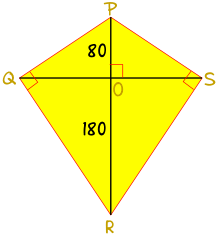### 例子：小山喜欢放风筝！

• 风筝的骨架是两根互相垂直的支杆，PR 和 QS，相交点是 O。
• PO = 80厘米，OR = 180厘米。
• 蒙面在 Q 与 S 是个直角。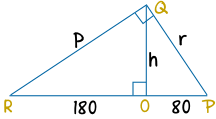h2 = 180 × 80 = 14400
h = √14400 = 120厘米

RP = RO + OP = 180厘米 + 80厘米 = 260厘米

r2 = 260 × 80 = 20800
r = √20800 = 144厘米（保留到最接近的厘米）

p2 = 260 × 180 = 46800
p = √46800 = 216厘米（保留到最近的厘米）